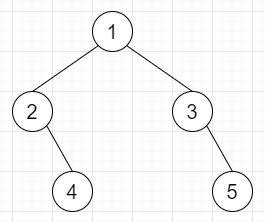# Cousins in Binary Tree in C++

Suppose we have a binary tree, the root node is present at depth 0, and children of each depth k node are at depth k+1.

Here two nodes of a binary tree are called cousins if they have the same depth, but have different parents.

All values of the tree will be unique, and the values x and y of two different nodes in the tree. We have to check whether the nodes corresponding to the values x and y are cousins or not.

So, if the input is likex = 5, y = 4, then the output will be true

To solve this, we will follow these steps −

• Define one map um

• Define one queue q

• insert root into q

• um[x] := um[y] := null

• while (not q is empty), do −

• qSize := size of q

• while qSize > 0 then (decrease qSize by 1), do −

• cur := first element of q

• delete element from q

• if left of curr is present, then −

• if um has value of left of cur, then

• um[value of left of cur] := cur

• Otherwise

• insert left of cur into q

• if um has value of right of cur, then

• um[value of right of cur] := cur

• Otherwise

• insert right of cur into q

• if um[x] or um[y] is non-zero, then −

• if um[x] is 0 or um[y] is 0 or um[x] is same as um[y], then −

• return false

• Otherwise

• return true

• return false

## Example

Let us see the following implementation to get a better understanding −

Live Demo

#include <bits/stdc++.h>
using namespace std;
class TreeNode {
public:
int val;
TreeNode *left, *right;
TreeNode(int data) {
val = data;
left = NULL;
right = NULL;
}
};
class Solution {
public:
bool isCousins(TreeNode *root, int x, int y) {
unordered_map<int, TreeNode *> um;
queue<TreeNode *> q;
q.push(root);
um[x] = um[y] = NULL;
while (!q.empty()) {
int qSize = q.size();
while (qSize-- > 0) {
auto cur = q.front();
q.pop();
if (cur->left && cur->left->val != 0)
if (um.count(cur->left->val))
um[cur->left->val] = cur;
else
q.push(cur->left);
if (cur->right && cur->right->val != 0)
if (um.count(cur->right->val))
um[cur->right->val] = cur;
else
q.push(cur->right);
}
if (um[x] or um[y])
if (!um[x] or !um[y] or um[x] == um[y])
return false;
else
return true;
}
return false;
}
};
main() {
Solution ob;
TreeNode *root = new TreeNode(1);
root->left = new TreeNode(2); root->right = new TreeNode(3);
root->left->right = new TreeNode(4); root->right->right = new TreeNode(5);
cout << (ob.isCousins(root, 5, 4));
}

## Input

TreeNode *root = new TreeNode(1);
root->left = new TreeNode(2); root->right = new TreeNode(3);
root->left->right = new TreeNode(4); root->right->right = new
TreeNode(5);
cout << (ob.isCousins(root, 5, 4));

## Output

1